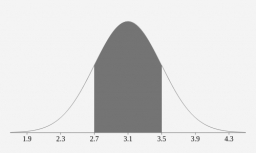# An exam - normal distribution

Five thousand students take an exam with a mean of 59 and a deviation of 8. How many students will score less than 75?

n2 =  4886

### Step-by-step explanation:Did you find an error or inaccuracy? Feel free to write us. Thank you!

Showing 1 comment:
Dr Math

Tips for related online calculators
Looking for help with calculating arithmetic mean?
Looking for a statistical calculator?
Looking for a standard deviation calculator?
Would you like to compute the count of combinations?

#### Grade of the word problem:

We encourage you to watch this tutorial video on this math problem: